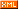# xuld

posts - 55, comments - 98, trackbacks - 0, articles - 2
::  ::  ::  ::::

## 1. 什么叫读懂代码

int fn(int a, int b){
int sum = 0;
for(int i = a; i < b; i++){
sum += fn(i - 1, i + 1);
}
return sum;
}

void fn(){
int a = f1();
int b = f2(a);
int c = f3(b);
int d = f4(a, b);
}

void fn(){
int value;
} else {
value = this.main;
}
}

## 3. 代码的歧义

function fn(){

}

function gn(){
fn();

// 这里是很长很长的代码。

function fn(){

}
}

[待续]